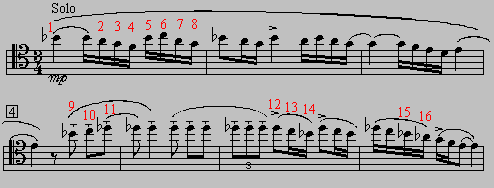Note numbers refer to the music below.

Ravel, Maurice. Bolero, opening solo. Groeling, Charles R. "Cultivating Bassoonists." The Instrumentalist 47/1 (August 1992): 52. For rapid Bb4-A4 combinations: A4 use Cooper p. 288 and for Bb4 use Cooper p. 291.

```
Note #2 A4:            Note #1 Bb4:
Eb                     Eb                                                     x x x | o o F          x x x | x x o F
a c#                   a c#```

Ravel, Maurice. Bolero, opening solo. Ledwith, Austin. In Klimko, Ronald. Bassoon Performance Practices and Teaching in the United States and Canada. Idaho: University of Idaho, 1974, p. 66.

```

Note # 11 Db5:
x o o | o o o
d```

Ravel, Maurice. Bolero, opening solo. Moritz, Frederick. "A Dissertation on the Use of Alternate Fingerings for Some of the High Register Notes on the Heckel and Heckel-Type Bassoons." The Double Reed 8/3 (Winter 1985): 34-37. Readers may find it most helpful to refer to the article itself. Fingerings for bassoons without the G# ring follow in order of first appearance in the solo:

First solo measure:

```

Note #1 Bb4:         Note #2 A4:
Eb                   Eb
x x x | x x o        x x x | o o x
a c#    E            a c#

Note # 3 G4:         Note #4 F4:
Eb                 Eb
1/4 x x | x o o F    x o x | x x o

Note# 6 C5:          Note #7 A4:
Eb                   Eb
x o o | o o o        / x x | o o x
b                   b```

Measure four of the solo. Use Bb4 (Note #9) and C5 (Note #10) given above, then

```Note #11 Db5:
Eb
x o x | x o x
E```

Measure six of the solo. Use Db5 (Note #12) and C5 (Note #13) given above, then

```Note # 14 Bb4:
Eb
x x x | x x o
b       E```

The fingerings for bassoons with the G# ring follow in order of appearance in the solo:

```Note #1 Bb4:        Note #2 A4:
Eb                  Eb
x x x | x x o F     x x x | o o o F
a c#                a c#

Note #3 G4:         Note #4 F4:
Eb                  Eb
/ x x | x o o G     x o x | x x

Note #6 C5:

x o o | o o o F
a c#```

Measure four of the solo. Use Bb4 given above, then

```Note #10 C5:          Note #11 Db5:
Eb                    Eb
x o o | o o o         x o x | x o x
b                     b      (E)```

Measure six of the solo. Use Db5 (Note #12) and the second C5 (Note #13) given above, then

```Note # 14 Bb4:
Eb
x x x | x x o
b       E```

Measure seven of the solo. Note #15 use second Bb4 then

```Note # 16 Ab4:
Eb
/ x x | o o o F```